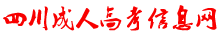﻿ 【江苏专升本】数学1--一元函数积分学知识点睛（定积分的应用）-江苏成人高考信息网# 【江苏专升本】数学1--一元函数积分学知识点睛（定积分的应用）

x=ax=b

y=f(x)，出来区域交的是y=f(x)

y=dy=c

x=j(y)，出来区域交的是x=j(y)y=f(x)y=f(x)x=ax=b

(1)画图.

(2)确定在x轴上的投影区间:[0,1].

(3)确定上下曲线:

(4)计算积分x=j(y)x=j(y)y=dy=c

(3)确定左右曲线x=j(y)x=j(y)

(1)画图

(2)确定在y轴上的投影区间：[-2,4]

(3)确定左右曲线

(4)计算积分：

### 江苏成考交流群### 点击加入考生微信群

#### 最新信息榜